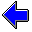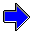Java Security Settings:
This web page employs Java, which requires specific security settings for correct operation.
If the applets on this page do not run correctly, consult the Virtual Chemistry Experiments FAQ
or the Physlet Physics web site for establishing the correct security settings.

# Calorimetry

## Heat of Combustion of Methane

### Concepts

The combustion reaction for methane is

CH4 (g) + 2 O2 (g)   →   CO2 (g) + 2 H2O (l)

The enthalpy change for this reaction is measured by pressurizing a strong metal reaction vessel (called a bomb) with a mixture of methane and oxygen gas. The bomb is immersed in a calorimeter filled with water. An electrical current is passed through ignition wire (a fine iron wire), which ignites the wire and the gas mixture.

The heat balance for this calorimetry experiment is:

0 = qcal + qwire + qcomb

The heat for the calorimeter, qcal, is determined from the heat capacity of the calorimeter and the temperature change for the calorimetry experiment. Typically the amount of water in the calorimeter is always the same; therefore Ccal includes the heat capacities of the calorimeter, the water, and the bomb itself.

The burning of the ignition wire releases heat, qwire, and this heat must be included in the calculations. (This heat is treated separately, because the amount of ignition wire used varies from one measurement to the next.)

The heat released by the combustion reaction is qcomb, which is related to the molar enthalpy of combustion by

 ΔHcomb = qcombnmethane

Combustion experiments are general conducted with a large excess of oxygen, so that the fuel (methane in this case) is the limiting reactant.

Combustion reactions are often used to calculate the molar enthalpies of formation. For example, the standard molar enthalpy of combustion for methane can be expressed in terms of the standard molar enthalpies of formation of the reactants and products:

ΔHocomb = 2 ΔHof,water + ΔHof,carbon dioxide - ΔHof,methane - 2 ΔHof,oxygen

ΔHocomb is measured experimentally.

ΔHof,oxygen = 0, because oxygen is a pure element.

The other molar enthalpies of formation are known from independent measurements. For example, one could determine the heat of combustion of hydrogen to obtain the molar enthalpy of formation for water.

For liquid water, ΔHof = -285.8 kJ mole-1

For gaseous carbon dioxide, ΔHof = -393.5 kJ mole-1

### Experiment

Objective:

• Determine the molar enthalpy of combustion for methane.
• Determine the molar enthalpy of formation for methane.

Approach:

• Pressurize the bomb with a known amount of methane gas.
• Observe the temperature of the system before and after the combustion reaction occurs.
• Calculate the change in temperature for the system.
• Use the temperature change, the heat capacity for the calorimeter, and the heat released by burning the ignition wire to calculate the heat of combustion.
• Divide the heat of neutralization by the moles of methane to determine the molar enthalpy of combustion.
• Use the molar enthalpy of combustion of methane to calculate the molar enthalpy of formation of methane.

### Part 1

In this part of the experiment, the calorimeter is filled with 10.0 mmole of methane gas and an excess of oxygen.

When burned, the ignition wire releases 107.2 J of heat

The heat capacity of the calorimeter (including the bomb and water) is 4.319 kJ oC-1.

### Part 2

Repeat the measurements made in Part 1 using a different initial pressure of methane gas in the bomb. As in Part 1, a large excess of oxygen is present.

Use the ideal gas law to calculate the amount of methane originally present.

The volume of the bomb is 271 mL.

The heat capacity of the calorimeter (including the bomb and water) is 4.319 kJ oC-1.

A different length of ignition wire is used in each experiment. The heat released by combustion of the ignition wire is shown at the right.

Pressure of
Methane
atm

Combustion of the Ignition Wire Releases
JHeat of Solution of Sulfuric Acid                     Heat of Solution of Calcium HydroxideCalorimetry Home PageVirtual Chemistry Home Page

HeatOfCombustionOfMethane.html version 2.1